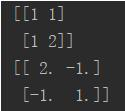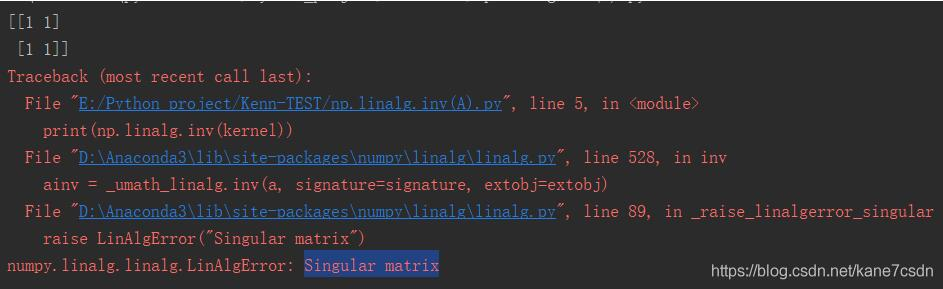• python矩阵求逆
千次阅读
2019-04-13 21:31:54

矩阵求逆
from numpy import *
a = mat([[0.6,-0.2,-0.1],[-0.1,0.5,-0.2],[-0.3,-0.4,0.8]])
b = a.I #大写的 i
# b 即为矩阵 a 的逆矩阵

更多相关内容
• 可用于求解希尔密码密钥的逆矩阵，根据逆矩阵与密文相乘再mod 26计算出明文
• ## python矩阵求逆

万次阅读 2018-11-25 09:58:36
mat = np.array( [[ 65.481, 128.553, 24.966 ], [-37.797, -74.203, 112.0 ], [ 112.0, -93.786, -18.214]]) mat_inv = np.linalg.inv(mat)#矩阵求逆 offset = np.array([16, 128, 128])
mat = np.array(
[[ 65.481, 128.553, 24.966 ],
[-37.797, -74.203, 112.0  ],
[  112.0, -93.786, -18.214]])
mat_inv = np.linalg.inv(mat)#矩阵求逆
offset = np.array([16, 128, 128])

展开全文tensorflow
• ## 09-30:Python矩阵求逆

千次阅读 2020-12-09 07:41:02
A的等于U的乘于L的，L的就利用下三角矩阵求逆算法进行求解，U的可以这样：先将U转置成下三角矩阵，再像对L求逆一样对U的转置求逆，再将得到的结果转置过来，得到的就是U的。因此，关键是下三角矩阵的...

旁听了今天的上机课，收获良多。

方阵A求逆，先做LU分解。A的逆等于U的逆乘于L的逆，L的逆就利用下三角矩阵求逆算法进行求解，U的逆可以这样求：先将U转置成下三角矩阵，再像对L求逆一样对U的转置求逆，再将得到的结果转置过来，得到的就是U的逆。

因此，关键是下三角矩阵的求逆。

1.下三角矩阵求逆算法

我利用的公式计算公式如下：对角元素.png对角元素以下的元素.png

我的代码如下：

def triInverse(matA):

'''

@author：zengwei

输入：

matA:一个等待求逆的下三角矩阵,大小为n*n，并且希望里面的元素值是浮点数

输出：

matInv:matA的逆矩阵

'''

numRows = matA.shape

matL = matA.copy()

matInv = np.zeros((numRows,numRows))

for row in np.arange(0,numRows):

matInv[row,row] = 1/matL[row,row]

for k in np.arange(row-1,-1,-1):

matInv[row,k] = -(np.dot(matInv[row,k+1:row+1],matL[k+1:row+1,k]))/matL[k,k]

return matInv

同样，生成一个矩阵来测试一下上面的函数，并得到输出。

import numpy as np

test_mat = np.array([[1,0,0,0],[2,3,0,0],[4,5,6,0],[7,8,9,10]],dtype=float)

'''

test_mat = array([[ 1., 0., 0., 0.],

[ 2., 3., 0., 0.],

[ 4., 5., 6., 0.],

[ 7., 8., 9., 10.]])

'''

Inv = triInverse(test_mat)

'''

Inv = array([[ 1. , 0. , 0. , 0. ],

[-0.66666667, 0.33333333, 0. , 0. ],

[-0.11111111, -0.27777778, 0.16666667, 0. ],

[-0.06666667, -0.01666667, -0.15 , 0.1 ]])

'''

显然，我不知道解出来对不对，但是我可以用这个逆矩阵Inv和测试矩阵test_mat相乘看看是不是单位矩阵来判断。

np.dot(test_mat,Inv)

'''

array([[1., 0., 0., 0.],

[0., 1., 0., 0.],

[0., 0., 1., 0.],

[0., 0., 0., 1.]])

'''

是单位矩阵，说明下三角矩阵求逆成功。接下来，利用上面的函数来进行矩阵的求逆。

2.矩阵求逆

首先，先贴出我LU分解的函数：

def getLandU(A):

'''

@author：zengwei

输入：

A:系数矩阵；array格式，且数值类型必须为浮点数，否则会出现截断误差。

输出：

LU分解中的L和U矩阵

'''

matA = A.copy()

if matA[0,0]==0:

print('不符合要求！需要换行操作。')

else:

numRows = matA.shape

matL = np.identity(numRows) # 准备给L矩阵用

for row in np.arange(0,numRows-1): # 前n-1行，row代表第几行

for k in range(row+1,numRows): # 在第row行的情况下，遍历剩下的行

matL[k,row] = matA[k,row]/matA[row,row] # 获得L矩阵

if matA[k,row] != 0:

matA[k,:]=matA[k,:] - (matA[k,row]/matA[row,row])*matA[row,:]

return matL,matA # matL为L矩阵，matA变为U矩阵

然后我们利用getLandU函数和triInverse函数来写矩阵求解函数。如下：

def matInverse(A):

'''

@author：zengwei

输入：

A:想要求逆的系数矩阵，n*n，希望里面的数值是浮点数

输出：

A的逆矩阵

'''

L,U = getLandU(A) #LU分解

L_inv = triInverse(L) #L求逆

U_inv = triInverse(U.T).T #U求逆

return np.dot(U_inv,L_inv)

下面，我们生成一个随机矩阵来测试，并验证求得的逆矩阵和原矩阵相乘是否为单位矩阵。

TestMat = np.float64(np.random.randint(0,10,(4,4)))

'''

TestMat = array([[3., 7., 4., 0.],

[8., 3., 9., 3.],

[5., 4., 2., 4.],

[7., 0., 7., 6.]])

'''

TestMatInv = matInverse(TestMat)

isI = np.int64(np.dot(TestMatInv,TestMat)) # 验证

'''

isI = array([[1, 0, 0, 0],

[0, 1, 0, 0],

[0, 0, 1, 0],

[0, 0, 0, 0]], dtype=int64)

'''

可见，逆矩阵乘与原矩阵是单位矩阵，这里用了一下np.int64()函数，是为了让结果好看。否则原本是0的地方就会是非常非常非常小的数，这是由于计算机用浮点数运算得到的效果。

放假。

展开全文• 我就废话不多说了，大家还是直接看代码吧~import numpy as npkernel = np.array([1, 1, 1, 2]).reshape((2, 2))print(kernel)print(np.linalg.inv(kernel))注意，Singular matrix奇异矩阵不可求逆补充：python+numpy...

我就废话不多说了，大家还是直接看代码吧~

import numpy as np

kernel = np.array([1, 1, 1, 2]).reshape((2, 2))

print(kernel)

print(np.linalg.inv(kernel))注意，Singular matrix奇异矩阵不可求逆补充：python+numpy中矩阵的逆和伪逆的区别

定义：

对于矩阵A，如果存在一个矩阵B，使得AB=BA=E，其中E为与A,B同维数的单位阵，就称A为可逆矩阵(或者称A可逆)，并称B是A的逆矩阵，简称逆阵。(此时的逆称为凯利逆)

矩阵A可逆的充分必要条件是|A|≠0。

伪逆矩阵是逆矩阵的广义形式。由于奇异矩阵或非方阵的矩阵不存在逆矩阵，但可以用函数pinv(A)求其伪逆矩阵。

基本语法为X=pinv(A),X=pinv(A,tol),其中tol为误差,pinv为pseudo-inverse的缩写：max(size(A))*norm(A)*eps。

函数返回一个与A的转置矩阵A' 同型的矩阵X，并且满足：AXA=A,XAX=X.此时，称矩阵X为矩阵A的伪逆，也称为广义逆矩阵。

pinv(A)具有inv(A)的部分特性，但不与inv(A)完全等同。

如果A为非奇异方阵，pinv(A)=inv(A)，但却会耗费大量的计算时间，相比较而言，inv(A)花费更少的时间。

代码如下：

1.矩阵求逆

import numpy as np

a = np.array([[1, 2], [3, 4]]) # 初始化一个非奇异矩阵(数组)

print(np.linalg.inv(a)) # 对应于MATLAB中 inv() 函数

# 矩阵对象可以通过 .I 求逆,但必须先使用matirx转化

A = np.matrix(a)

print(A.I)

2.矩阵求伪逆

import numpy as np

# 定义一个奇异阵 A

A = np.zeros((4, 4))

A[0, -1] = 1

A[-1, 0] = -1

A = np.matrix(A)

print(A)

# print(A.I) 将报错，矩阵 A 为奇异矩阵，不可逆

print(np.linalg.pinv(A)) # 求矩阵 A 的伪逆(广义逆矩阵)，对应于MATLAB中 pinv() 函数

这就是矩阵的逆和伪逆的区别

截至2020/10/4，matrix函数还可以使用，但已经过时，应该是mat函数这种。

以上为个人经验，希望能给大家一个参考，也希望大家多多支持我们。如有错误或未考虑完全的地方，望不吝赐教。

时间： 2021-03-09

展开全文• python矩阵求逆及矩阵相乘，求解，和矩阵转化等有关矩阵算法
• 先吐槽两句，真的是Matlab才不会报这种错，今天计算逆矩阵报了个这么个错，一个简单的2*2的可逆矩阵居然死活不出来，好气啊。 TypeError: No loop matching the specified signature and casting was found for ...TypeError astype 数据类型转换
• python,c矩阵求逆问题记录 目录 python,c矩阵求逆问题记录 前言 正文 现象 优化思路 最终方案 结束语 前言 记录下自己在做相机矫正遇到的问题，详细说下就是np.linalg.inv(M) 和cv2.invert(M)的结果居然不一样。...opencv 图像处理 android
• import numpy as np A = np.matrix([[1,2,3],[4,5,6],[7,8,9]]) B = np.array([1,2,3,4,5,6,7,8,9]).reshape((3,3)) print(A) print(B) print("*" * 50) print(np.linalg.inv(A)) print(np.linalg.inv(B)) ...
• ## Python中求矩阵的逆

万次阅读 2018-11-26 19:48:25
import numpy as np kernel = np.array([1, 1, 1, 2]).reshape((2, 2)) print(kernel) print(np.linalg.inv(kernel)) 注意，Singular matrix奇异矩阵不可求逆
• 使用Continuum Anaconda分布中的Sympy 0.7.2(我懒得自己构建堆栈，不要讨厌)，当我在做复杂矩阵代数时，经常遇到这个问题，其中一些本机函数将典型的“1j”替换为“I”。这很烦人，因为它不能很好地处理其他算术运算...
• #模26运算下，求矩阵。 #使用伴随矩阵求逆矩阵法。 #整个精度保持在整数位（方便模运算）。 import numpy as np list_A1 = [] #目标矩阵A1 A1 = np.array([[3,13,21,9],[15,10,6,25],[10,17,4,8],[1,23,7,...numpy
• 前提是不使用numpy等库，自己编写求矩阵，当一个比较小的矩阵时，结果是正确的，但当矩阵一大(3000*60)，便出现与用numpy算出来结果不一样了，不知道是不是精度问题，该如何改进？# 本函数求矩阵def ...
• import sympy from sympy import * t= symbols('t') px1, py1, pz1, vx1, vy1, vz1, ax1, ay1, az1 = symbols('px1 py1 pz1 vx1 vy1 vz1 ax1 ay1 az1') px2, py2, pz2, vx2, vy2, vz2, ax2, ay2, az2 = symbols('px2...
• 本文实例讲述了python简单实现矩阵的乘，加，转置和运算。分享给大家供大家参考，具体如下：使用python完成矩阵的乘，加，转置和：# -*- coding:utf-8 -*-#矩阵的乘，加，转置和#numpy库提供矩阵运算的功能...
• 主要介绍了python矩阵运算,转置,运算,共轭矩阵实例，具有很好的参考价值，希望对大家有所帮助。一起跟随小编过来看看吧
• 我在python中做矩阵求逆，结果因数据规模不同而不同，这很奇怪。在在下面的代码中，预计A_inv/B_inv=B/A，但它表明A_inv/B_inv与B/A之间的差异随着数据规模的增大而越来越大。。。这是因为Python不能精确计算大值...
• python求逆矩阵库 https://numpy.org/ C++求逆矩阵库 http://eigen.tuxfamily.org/index.php?title=Main_Page
• 在进行处理数据时，我们总是需要对矩阵进行操作。比如说矩阵的转置，求逆等等。但是，我发现网上的一些帖子似乎对于这个问题的解答有些错误，因此，我想分享一些我对此问题的看法。numpy
• 假定有方阵A， A 的逆矩阵Python 中使用 numpy 库函数命令实现。 import numpy as np A_1 = np.linalg.inv(A) # 逆矩阵（奇异矩阵没有逆矩阵，不可以用此命令计算） A_2 = np.linalg.pinv(A) # 伪逆矩阵...开发语言 后端
• 原理：应用列主元消去法运算矩阵A的逆矩阵，利用初等矩阵行变换A转化单位矩阵时，同样的行变化可将单位矩阵转化为A的逆矩阵。 步骤： 编制下三角部分消元和上三角部分消元的代码 a. 从对角线元素往下比较取得这...
• ## Numpy 中的矩阵求逆

万次阅读 多人点赞 2019-02-01 12:37:02
1. 矩阵求逆 import numpy as np a = np.array([[1, 2], [3, 4]]) # 初始化一个非奇异矩阵 print(a.I) print(np.linalg.inv(a)) #与上一步等同，对应于MATLAB中 inv() 函数 2. 矩阵求 import numpy as np # ...Numpy
• ## 奇异矩阵-python

千次阅读 2020-12-10 18:53:38
下面的代码显示了一个矩阵的奇点问题，因为我在Pycharm中工作raise LinAlgError("Singular matrix")numpy.linalg.linalg.LinAlgError: Singular matrix我想问题是K，但我不能确切地理解如何：from numpy import ......